Ohm's Law Ultra Calculator Scroll down for instructions.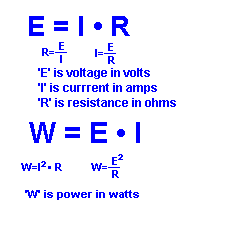Click on the two quantities you know: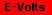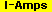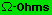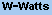I N S T R U C T I O N S

If you know any two of these four quantities:
1) volts 2) amps 3) ohms 4) watts
this calculator will solve for the other two.
You can input three types of unit for each quantity.
The answers will be displayed in three types of unit for each quantity.

E X A M P L E S
 1) A 15 volt circuit has a current of 200 milliamps. What is the resistance? First select the two known quantities. In this case, we know voltage (E) and current (I) so click on the "E+I" button.Next, click on the appropriate units which are 'volts' and 'milliamps'. Enter the numbers and then click 'calculate'. The answer is 75 ohms, .075 kilohms and .000075 megohms and you also get the wattage expressed in 3 different units.
2) A 120 volt, 25 watt refrigerator bulb is using how much current?

Since you know the voltage (E) and power (W), click on the "E+W" button, then click on volts and milliamps, then enter the numbers.
It is using 208.33 milliamps and even though it wasn't asked, the resistance is 576 ohms.

3) 275 milliamps is flowing through a 200 microwatt circuit. What is the voltage?

You know the current (I) and power (W), so click on the "I + W" button, then click on milliamps and watts, enter the numbers and then click calculate.
The circuit voltage is 727.27 microvolts and the resistance is .0026446 ohms.

Significant Figures>>>

The default numeric display is 5 significant figures. For easier readability, numbers that are greater than .001 and less than 1000 are NOT in scientific notation. You can change the display option by changing the number in the box above. Entering a zero eliminates all formatting.